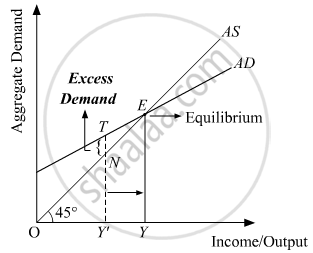# Answer the Following Question. Describe the Adjustments that May Take Place in an Economy When Ex-ante Aggregate Demand is Greater than Ex-ante Aggregate Supply. - Economics

Describe the adjustments that may take place in an economy when ex-ante Aggregate Demand is greater than ex-ante Aggregate Supply.

#### Solution

In case, if AD > AS, then it implies a situation, where the total demand for goods and services is more than the total supply of goods and services. This implies a situation of excess demand. Due to the excess demand, the producers draw down their inventory and increase production. The increase in production requires hiring more factors of production, thereby increases employment level and income. Finally, the income will rise sufficiently to equate the AD with AS, thus the equilibrium is restored back. This process of adjustment mechanism is explained below graphically.In the figure, AD and AS represent the aggregate demand and aggregate supply curves. Let us suppose that the equilibrium is operating at a situation, where aggregate demand exceeds aggregate supply, i.e. AD > AS. TY' represents the aggregate demand of output by the economy but the aggregate supply is only of NY'. Hence, the economy is facing excess demand equivalent to TN (i.e. TY' – NY'). Due to the excess demand, the producers draw down their inventories and hire more factors of production. This results in an increase in production and employment. The income, output, and employment will continue to rise until all the excess demand is wiped-out. This happens at equilibrium point E, where AD and AS intersect each other. At the equilibrium, OY represents the equilibrium level of output.

Concept: Concept of Aggregate Demand and Aggregate Supply
Is there an error in this question or solution?